# 8.3 Add and subtract rational expressions with a common denominator  (Page 2/2)

 Page 2 / 2

Subtract: $\frac{{x}^{2}}{x+3}-\frac{9}{x+3}.$

$x-3$

Subtract: $\frac{4{x}^{2}}{2x-5}-\frac{25}{2x-5}.$

$2x+5$

Be careful of the signs when you subtract a binomial!

Subtract: $\frac{{y}^{2}}{y-6}-\frac{2y+24}{y-6}.$

## Solution

$\begin{array}{cccc}& & & \hfill \phantom{\rule{5em}{0ex}}\frac{{y}^{2}}{y-6}-\frac{2y+24}{y-6}\hfill \\ \\ \\ \begin{array}{c}\text{The fractions have a common}\hfill \\ \text{denominator, so subtract the numerators}\hfill \\ \text{and place the difference over the}\hfill \\ \text{common denominator.}\hfill \end{array}\hfill & & & \hfill \phantom{\rule{5em}{0ex}}\frac{{y}^{2}-\left(2y+24\right)}{y-6}\hfill \\ \\ \\ \text{Distribute the sign in the numerator.}\hfill & & & \hfill \phantom{\rule{5em}{0ex}}\frac{{y}^{2}-2y-24}{y-6}\hfill \\ \\ \\ \text{Factor the numerator.}\hfill & & & \hfill \phantom{\rule{5em}{0ex}}\frac{\left(y-6\right)\left(y+4\right)}{y-6}\hfill \\ \\ \\ \text{Remove common factors.}\hfill & & & \hfill \phantom{\rule{5em}{0ex}}\frac{\overline{)\left(y-6\right)}\left(y+4\right)}{\overline{)y-6}}\hfill \\ \\ \\ \text{Simplify.}\hfill & & & \hfill \phantom{\rule{5em}{0ex}}y+4\hfill \end{array}$

Subtract: $\frac{{n}^{2}}{n-4}-\frac{n+12}{n-4}.$

$n+3$

Subtract: $\frac{{y}^{2}}{y-1}-\frac{9y-8}{y-1}.$

$y-8$

Subtract: $\frac{5{x}^{2}-7x+3}{{x}^{2}-3x+18}-\frac{4{x}^{2}+x-9}{{x}^{2}-3x+18}.$

## Solution

$\begin{array}{cccc}& & & \hfill \phantom{\rule{5em}{0ex}}\frac{5{x}^{2}-7x+3}{{x}^{2}-3x+18}-\frac{4{x}^{2}+x-9}{{x}^{2}-3x+18}\hfill \\ \\ \\ \begin{array}{c}\text{Subtract the numerators and place the}\hfill \\ \text{difference over the common}\hfill \\ \text{denominator.}\hfill \end{array}\hfill & & & \hfill \phantom{\rule{5em}{0ex}}\frac{5{x}^{2}-7x+3-\left(4{x}^{2}+x-9\right)}{{x}^{2}-3x+18}\hfill \\ \\ \\ \text{Distribute the sign in the numerator.}\hfill & & & \hfill \phantom{\rule{5em}{0ex}}\frac{5{x}^{2}-7x+3-4{x}^{2}-x+9}{{x}^{2}-3x-18}\hfill \\ \\ \\ \text{Combine like terms.}\hfill & & & \hfill \phantom{\rule{5em}{0ex}}\frac{{x}^{2}-8x+12}{{x}^{2}-3x-18}\hfill \\ \\ \\ \begin{array}{c}\text{Factor the numerator and the}\hfill \\ \text{denominator.}\hfill \end{array}\hfill & & & \hfill \phantom{\rule{5em}{0ex}}\frac{\left(x-2\right)\left(x-6\right)}{\left(x+3\right)\left(x-6\right)}\hfill \\ \\ \\ \text{Simplify by removing common factors.}\hfill & & & \hfill \phantom{\rule{5em}{0ex}}\frac{\left(x-2\right)\overline{)\left(x-6\right)}}{\left(x+3\right)\overline{)\left(x-6\right)}}\hfill \\ \text{Simplify.}\hfill & & & \hfill \phantom{\rule{5em}{0ex}}\frac{\left(x-2\right)}{\left(x+3\right)}\hfill \end{array}$

Subtract: $\frac{4{x}^{2}-11x+8}{{x}^{2}-3x+2}-\frac{3{x}^{2}+x-3}{{x}^{2}-3x+2}.$

$\frac{x-11}{x-2}$

Subtract: $\frac{6{x}^{2}-x+20}{{x}^{2}-81}-\frac{5{x}^{2}+11x-7}{{x}^{2}-81}.$

$\frac{x-3}{x+9}$

## Add and subtract rational expressions whose denominators are opposites

When the denominators of two rational expressions are opposites, it is easy to get a common denominator. We just have to multiply one of the fractions by $\frac{-1}{-1}$ .

Let’s see how this works.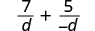Multiply the second fraction by $\frac{-1}{-1}$ .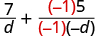The denominators are the same.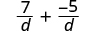Simplify.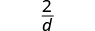Add: $\frac{4u-1}{3u-1}+\frac{u}{1-3u}.$

## Solution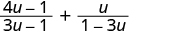The denominators are opposites, so multiply the second fraction by $\frac{-1}{-1}$ .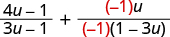Simplify the second fraction.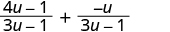The denominators are the same. Add the numerators.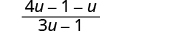Simplify.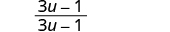Simplify.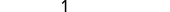Add: $\frac{8x-15}{2x-5}+\frac{2x}{5-2x}.$

$3$

Add: $\frac{6{y}^{2}+7y-10}{4y-7}+\frac{2{y}^{2}+2y+11}{7-4y}.$

$y+3$

Subtract: $\frac{{m}^{2}-6m}{{m}^{2}-1}-\frac{3m+2}{1-{m}^{2}}.$

## Solution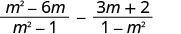The denominators are opposites, so multiply the second fraction by $\frac{-1}{-1}$ .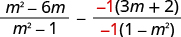Simplify the second fraction.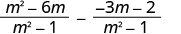The denominators are the same. Subtract the numerators.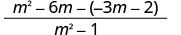Distribute. $\frac{{m}^{2}-6m+3m+2}{{m}^{2}-1}$ Combine like terms.Factor the numerator and denominator.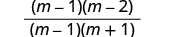Simplify by removing common factors.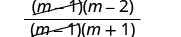Simplify.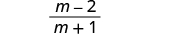Subtract: $\frac{{y}^{2}-5y}{{y}^{2}-4}-\frac{6y-6}{4-{y}^{2}}.$

$\frac{y+3}{y+2}$

Subtract: $\frac{2{n}^{2}+8n-1}{{n}^{2}-1}-\frac{{n}^{2}-7n-1}{1-{n}^{2}}.$

$\frac{3n-2}{n-1}$

## Key concepts

• If $p,q,\phantom{\rule{0.2em}{0ex}}\text{and}\phantom{\rule{0.2em}{0ex}}r$ are polynomials where $r\ne 0$ , then
$\frac{p}{r}+\frac{q}{r}=\frac{p+q}{r}$
• To add rational expressions with a common denominator, add the numerators and place the sum over the common denominator.
• Rational Expression Subtraction
• If $p,q,\phantom{\rule{0.2em}{0ex}}\text{and}\phantom{\rule{0.2em}{0ex}}r$ are polynomials where $r\ne 0$ , then
$\frac{p}{r}-\frac{q}{r}=\frac{p-q}{r}$
• To subtract rational expressions, subtract the numerators and place the difference over the common denominator.

## Practice makes perfect

Add Rational Expressions with a Common Denominator

$\frac{2}{15}+\frac{7}{15}$

$\frac{3}{5}$

$\frac{4}{21}+\frac{3}{21}$

$\frac{7}{24}+\frac{11}{24}$

$\frac{3}{4}$

$\frac{7}{36}+\frac{13}{36}$

$\frac{3a}{a-b}+\frac{1}{a-b}$

$\frac{3a+1}{a+b}$

$\frac{3c}{4c-5}+\frac{5}{4c-5}$

$\frac{d}{d+8}+\frac{5}{d+8}$

$\frac{d+5}{d+8}$

$\frac{7m}{2m+n}+\frac{4}{2m+n}$

$\frac{{p}^{2}+10p}{p+2}+\frac{16}{p+2}$

$p+8$

$\frac{{q}^{2}+12q}{q+3}+\frac{27}{q+3}$

$\frac{2{r}^{2}}{2r-1}+\frac{15r-8}{2r-1}$

$r+8$

$\frac{3{s}^{2}}{3s-2}+\frac{13s-10}{3s-2}$

$\frac{8{t}^{2}}{t+4}+\frac{32t}{t+4}$

$8t$

$\frac{6{v}^{2}}{v+5}+\frac{30v}{v+5}$

$\frac{2{w}^{2}}{{w}^{2}-16}+\frac{8w}{{w}^{2}-16}$

$\frac{2w}{w-4}$

$\frac{7{x}^{2}}{{x}^{2}-9}+\frac{21x}{{x}^{2}-9}$

Subtract Rational Expressions with a Common Denominator

In the following exercises, subtract.

$\frac{{y}^{2}}{y+8}-\frac{64}{y+8}$

$y-8$

$\frac{{z}^{2}}{z+2}-\frac{4}{z+2}$

$\frac{9{a}^{2}}{3a-7}-\frac{49}{3a-7}$

$3a+7$

$\frac{25{b}^{2}}{5b-6}-\frac{36}{5b-6}$

$\frac{{c}^{2}}{c-8}-\frac{6c+16}{c-8}$

$c+2$

$\frac{{d}^{2}}{d-9}-\frac{6d+27}{d-9}$

$\frac{3{m}^{2}}{6m-30}-\frac{21m-30}{6m-30}$

$\frac{m-2}{3}$

$\frac{2{n}^{2}}{4n-32}-\frac{30n-16}{4n-32}$

$\frac{6{p}^{2}+3p+4}{{p}^{2}+4p-5}-\frac{5{p}^{2}+p+7}{{p}^{2}+4p-5}$

$\frac{p+3}{p+5}$

$\frac{5{q}^{2}+3q-9}{{q}^{2}+6q+8}-\frac{4{q}^{2}+9q+7}{{q}^{2}+6q+8}$

$\frac{5{r}^{2}+7r-33}{{r}^{2}-49}-\frac{4{r}^{2}-5r-30}{{r}^{2}-49}$

$\frac{r+9}{r+7}$

$\frac{7{t}^{2}-t-4}{{t}^{2}-25}-\frac{6{t}^{2}+2t-1}{{t}^{2}-25}$

Add and Subtract Rational Expressions whose Denominators are Opposites

$\frac{10v}{2v-1}+\frac{2v+4}{1-2v}$

$4$

$\frac{20w}{5w-2}+\frac{5w+6}{2-5w}$

$\frac{10{x}^{2}+16x-7}{8x-3}+\frac{2{x}^{2}+3x-1}{3-8x}$

$x+2$

$\frac{6{y}^{2}+2y-11}{3y-7}+\frac{3{y}^{2}-3y+17}{7-3y}$

In the following exercises, subtract.

$\frac{{z}^{2}+6z}{{z}^{2}-25}-\frac{3z+20}{25-{z}^{2}}$

$\frac{z+4}{z-5}$

$\frac{{a}^{2}+3a}{{a}^{2}-9}-\frac{3a-27}{9-{a}^{2}}$

$\frac{2{b}^{2}+30b-13}{{b}^{2}-49}-\frac{2{b}^{2}-5b-8}{49-{b}^{2}}$

$\frac{4b-3}{b-7}$

$\frac{{c}^{2}+5c-10}{{c}^{2}-16}-\frac{{c}^{2}-8c-10}{16-{c}^{2}}$

## Everyday math

Sarah ran 8 miles and then biked 24 miles. Her biking speed is 4 mph faster than her running speed. If $r$ represents Sarah’s speed when she ran, then her running time is modeled by the expression $\frac{8}{r}$ and her biking time is modeled by the expression $\frac{24}{r+4}.$ Add the rational expressions $\frac{8}{r}+\frac{24}{r+4}$ to get an expression for the total amount of time Sarah ran and biked.

$\frac{32r+32}{r\left(r+4\right)}$

If Pete can paint a wall in $p$ hours, then in one hour he can paint $\frac{1}{p}$ of the wall. It would take Penelope 3 hours longer than Pete to paint the wall, so in one hour she can paint $\frac{1}{p+3}$ of the wall. Add the rational expressions $\frac{1}{p}+\frac{1}{p+3}$ to get an expression for the part of the wall Pete and Penelope would paint in one hour if they worked together.

## Writing exercises

Donald thinks that $\frac{3}{x}+\frac{4}{x}$ is $\frac{7}{2x}.$ Is Donald correct? Explain.

Explain how you find the Least Common Denominator of ${x}^{2}+5x+4$ and ${x}^{2}-16.$

## Self check

After completing the exercises, use this checklist to evaluate your mastery of the objectives of this section.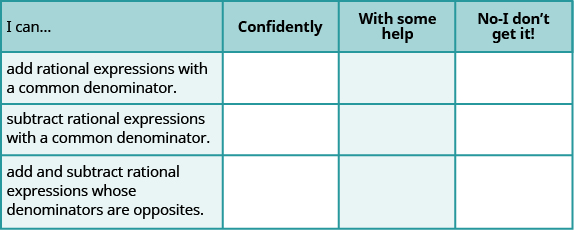What does this checklist tell you about your mastery of this section? What steps will you take to improve?

Aziza is solving this equation-2(1+x)=4x+10
No. 3^32 -1 has exactly two divisors greater than 75 and less than 85 what is their product?
x^2+7x-19=0 has Two solutions A and B give your answer to 3 decimal places
3. When Jenna spent 10 minutes on the elliptical trainer and then did circuit training for20 minutes, her fitness app says she burned 278 calories. When she spent 20 minutes onthe elliptical trainer and 30 minutes circuit training she burned 473 calories. How manycalories does she burn for each minute on the elliptical trainer? How many calories doesshe burn for each minute of circuit training?
.473
Angelita
?
Angelita
John left his house in Irvine at 8:35 am to drive to a meeting in Los Angeles, 45 miles away. He arrived at the meeting at 9:50. At 3:30 pm, he left the meeting and drove home. He arrived home at 5:18.
p-2/3=5/6 how do I solve it with explanation pls
P=3/2
Vanarith
1/2p2-2/3p=5p/6
James
Cindy
4.5
Ruth
is y=7/5 a solution of 5y+3=10y-4
yes
James
Cindy
Lucinda has a pocketful of dimes and quarters with a value of $6.20. The number of dimes is 18 more than 3 times the number of quarters. How many dimes and how many quarters does Lucinda have? Rhonda Reply Find an equation for the line that passes through the point P ( 0 , − 4 ) and has a slope 8/9 . Gabriel Reply is that a negative 4 or positive 4? Felix y = mx + b Felix if negative -4, then -4=8/9(0) + b Felix -4=b Felix if positive 4, then 4=b Felix then plug in y=8/9x - 4 or y=8/9x+4 Felix Macario is making 12 pounds of nut mixture with macadamia nuts and almonds. macadamia nuts cost$9 per pound and almonds cost $5.25 per pound. how many pounds of macadamia nuts and how many pounds of almonds should macario use for the mixture to cost$6.50 per pound to make?
Nga and Lauren bought a chest at a flea market for $50. They re-finished it and then added a 350 % mark - up Makaila Reply$1750
Cindy
the sum of two Numbers is 19 and their difference is 15
2, 17
Jose
interesting
saw
4,2
Cindy
Felecia left her home to visit her daughter, driving 45mph. Her husband waited for the dog sitter to arrive and left home 20 minutes, or 13 hour later. He drove 55mph to catch up to Felecia. How long before he reaches her?
hola saben como aser un valor de la expresión
NAILEA
integer greater than 2 and less than 12
2 < x < 12
Felix
I'm guessing you are doing inequalities...
Felix
Actually, translating words into algebraic expressions / equations...
Felix
hi
Darianna
hello
Mister
Eric here
Eric
6
Cindy

#### Get Jobilize Job Search Mobile App in your pocket Now!By Robert MurphyByBy Madison ChristianBy OpenStaxBy Dewey ComptonBy OpenStaxBy Michael PittBy OpenStaxBy Jazzycazz JacksonBy